• 提出了一种仅基于单个模板的人脸仿射配准方法。首先, 为了克服人脸仿射变换而产生的局部形变, 引入颜色特征来平衡模板人脸和目标人脸之间的颜色相似性和形状错配率, 进而提出了一种基于颜色特征的人脸粗搜索算法。...
• ## 仿射变换（Affine transformation）

万次阅读 多人点赞 2018-05-29 16:58:34
一个集合 XXX 的仿射变换为： f(x)=Ax+b,x∈Xf(x)=Ax+b,x∈Xf(x)=Ax+b, \quad x\in X 它的几何意义是对一个图形进行： 缩放（Scale）、平移(transform)、旋转(rotate)、反射（reflection, 对图形照镜子）、错切...
一个集合 $X$ 的仿射变换为：
$f(x)=Ax+b, \quad x\in X$
它的几何意义是对一个图形进行：
缩放（Scale）、平移(translate)、旋转(rotate)、反射（reflection, 对图形照镜子）、错切(shear mapping，感觉像是一个图形的倒影)
或者它们的任意组合
（$Ax$加上向量  $b$，相当于对 $Ax$ 平移）
维基百科中的一个图很好诠释了各种仿射变换：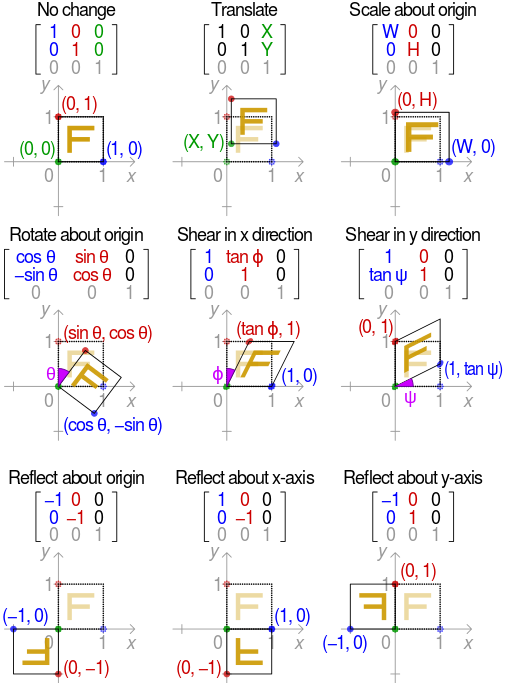仿射变换中集合中的一些性质保持不变：
（1）凸性
（2）共线性：若几个点变换前在一条线上，则仿射变换后仍然在一条线上
（3）平行性：若两条线变换前平行，则变换后仍然平行
（4）共线比例不变性：变换前一条线上两条线段的比例，在变换后比例仍然步
注：所有的三角形都能通过仿射变化为其他三角形，所有平行四边形也能仿射变换为另一个平行四边形。


展开全文• 我们通过利用仿射Poisson组的结构来概括Poisson-Lie T对偶，这是前一段时间在Poisson几何学中引入的概念，是对Poisson-Lie组的推广。 我们还引入了一个仿射拟泊松群的新概念，并表明它引起了一个更为通用的T对偶框架...
• 仿射变换： 从 到 的映射 ，称为仿射变换（affine transform）或仿射映射（affine map），其中 A 是一个 矩阵，b 是一个 m 维向量，x 是一个n维向量。 经过仿射变换，x从一个n维向量变成了一个m维向量。 仿射...
仿射变换：

从到的映射，称为仿射变换（affine transform）或仿射映射（affine map），其中 A 是一个矩阵，b 是一个 m 维向量，x 是一个n维向量。 经过仿射变换，x从一个n维向量变成了一个m维向量。

仿射函数：

当m==1时，上述仿射变换称为仿射函数。即仿射函数的作用是把一个n维向量变成一个实数。
展开全文• 仿射变换2. 旋转2.1 demo3. 透视变换3.1 demo4. 弯曲4.1 S形状4.2 波浪形5. 鱼眼特效6. 结构定义结构定义（中文）参考文档 1. 仿射变换 可将图片变换为平行四边形 /**@brief从三对对应点计算仿射变换。 @param src...
文章目录1. 仿射变换2. 旋转2.1 demo3. 透视变换3.1 demo4. 弯曲4.1 S形状4.2 波浪形5. 鱼眼特效6. 结构定义结构定义（中文）参考文档
1. 仿射变换
可将图片变换为平行四边形

/**@brief从三对对应点计算仿射变换。
@param src[] :原图上取三点坐标（三角形）
@param dst[] :目标三点坐标
@param return :返回2*3的变换矩阵
*/
CV_EXPORTS Mat getAffineTransform( const Point2f src[], const Point2f dst[] );

/ ** @brief对图像应用仿射变换。
函数warpAffine使用指定的矩阵转换源图像：
@param src输入图像。
@param dst输出图像，其大小为dsize，并且与src类型相同。
@param M \ f $2 \ times 3 \ f$转换矩阵。
@param dsize输出图像的大小。
@param标志插值方法( 详见 结构定义)
@param borderMode像素外推方法（ 详见 结构定义）
borderMode =＃BORDER_TRANSPARENT，表示目标图像中的像素对应于源图像中的“异常值”不会被该功能修改。
@param borderValue边界不变时使用的值；默认情况下为0。

@sa warpPerspective，调整大小，重新映射，getRectSubPix，转换
*/
CV_EXPORTS_W void warpAffine( InputArray src, OutputArray dst,
InputArray M, Size dsize,
int flags = INTER_LINEAR,
int borderMode = BORDER_CONSTANT,
const Scalar& borderValue = Scalar());

2. 旋转
/ ** @brief计算2D旋转的仿射矩阵。
@param center源图像中旋转的中心。
@param angle旋转角度，以度为单位。 正值表示逆时针旋转（坐标原点假定为左上角）。
@param scale各向同性比例因子。
@sa getAffineTransform，warpAffine，变换
* /
CV_EXPORTS_W Mat getRotationMatrix2D( Point2f center, double angle, double scale );

2.1 demo
int MPT_test_warpAffine(std::string file)
{
Point2f srcTri;
Point2f dstTri;
Mat rot_mat(2, 3, CV_32FC1);
Mat warp_mat(2, 3, CV_32FC1);
Mat src, warp_dst, warp_rotate_dst;

// 加载源图像
// 设置目标图像的大小和类型与源图像一致
warp_dst = Mat::zeros(src.rows, src.cols, src.type());
// 设置源图像和目标图像上的三组点以计算仿射变换
srcTri = cv::Point2f(0, 0);
srcTri = cv::Point2f(src.cols - 1, 0);
srcTri = cv::Point2f(0, src.rows - 1);
dstTri = cv::Point2f(0, 0);
dstTri = cv::Point2f(src.cols - 1, src.rows * 0.5);
dstTri = cv::Point2f(0, src.rows * 0.5);

// 求得仿射变换
warp_mat = getAffineTransform(srcTri, dstTri);
// 对源图像应用上面求得的仿射变换
warpAffine(src, warp_dst, warp_mat, warp_dst.size());
/** 对图像扭曲后再旋转 */
// 计算绕图像中点顺时针旋转50度缩放因子为0.6的旋转矩阵
Point center = Point(warp_dst.cols / 2, warp_dst.rows / 2);
double angle = -50.0;
double scale = 0.6;
// 通过上面的旋转细节信息求得旋转矩阵
rot_mat = getRotationMatrix2D(center, angle, scale);
// 旋转已扭曲图像
warpAffine(warp_dst, warp_rotate_dst, rot_mat, warp_dst.size());
// 显示结果
namedWindow("src", CV_WINDOW_NORMAL);
namedWindow("warp", CV_WINDOW_NORMAL);
namedWindow("warp_rotate", CV_WINDOW_NORMAL);
imshow("src", src);
imshow("warp", warp_dst);
imshow("warp_rotate", warp_rotate_dst);
waitKey(0);
return 0;
}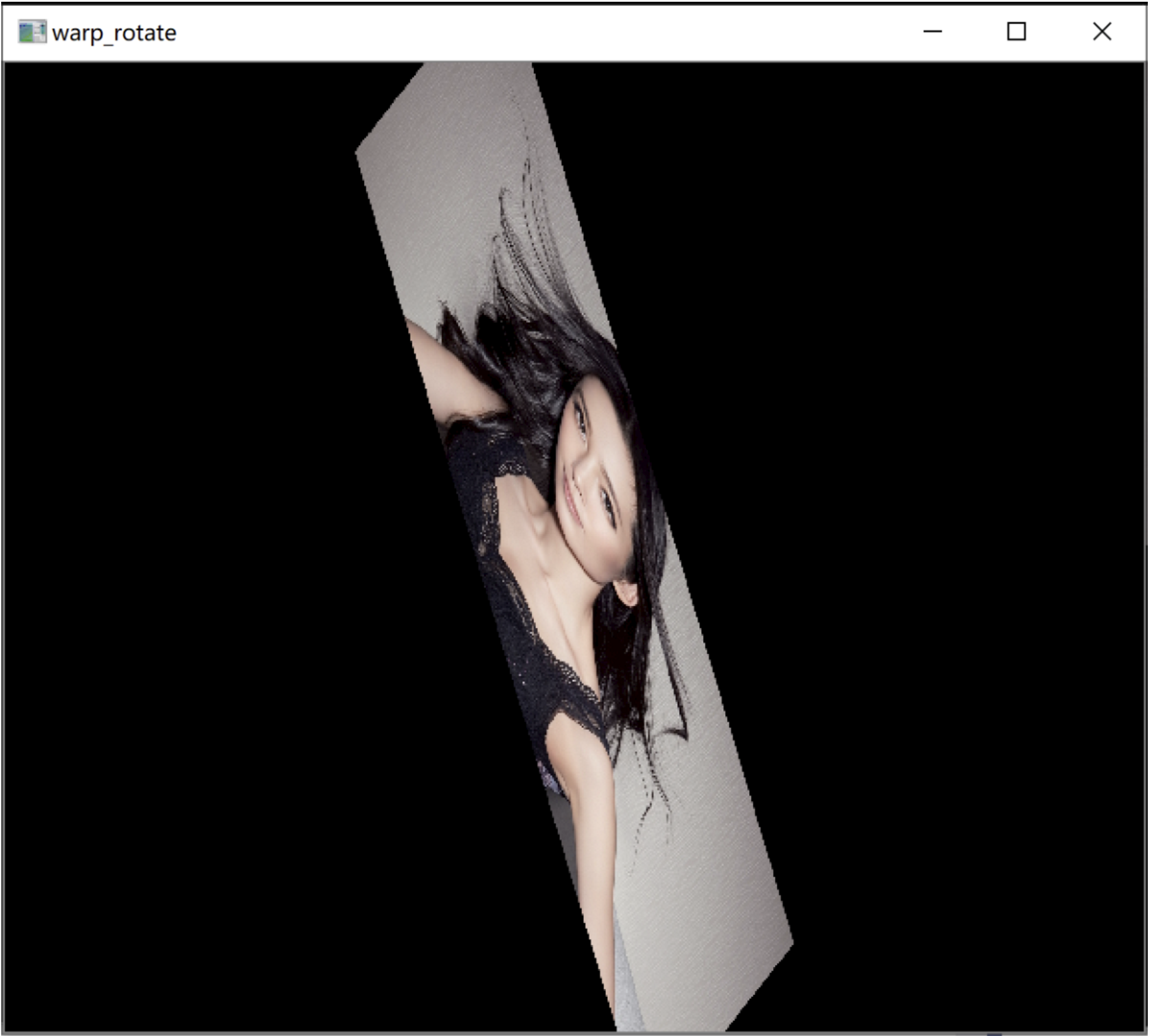3. 透视变换
可将矩形图片变换成梯形

根据4组点变换 返回3*3矩阵
CV_EXPORTS Mat getPerspectiveTransform( const Point2f src[], const Point2f dst[] );

/ ** @brief将透视转换应用于图像。
@param src输入图像。
@param dst输出图像，其大小为dsize，并且与src类型相同。
@param M \ f $3 \ times 3 \ f$转换矩阵。
@param dsize输出图像的大小。
@param标志插值方法
@param borderMode像素外推方法（#BORDER_CONSTANT或#BORDER_REPLICATE）。
@param borderValue边界不变时使用的值；默认情况下，它等于0。
@sa warpAffine，调整大小，重新映射，getRectSubPix，perspectiveTransform
* /
CV_EXPORTS_W void warpPerspective( InputArray src, OutputArray dst,
InputArray M, Size dsize,
int flags = INTER_LINEAR,
int borderMode = BORDER_CONSTANT,
const Scalar& borderValue = Scalar());

3.1 demo
int MPT_test_warpPerspective(std::string file)
{
Point2f srcTri;
Point2f dstTri;
Mat warpPerspective_mat(3, 3, CV_32FC1);
Mat src, warpPerspective_dst;

// Set the dst image the same type and size as src
warpPerspective_dst = Mat::zeros(src.rows, src.cols, src.type());

// 设置四组点，求出变换矩阵
srcTri = Point2f(0, 0);
srcTri = Point2f(src.cols - 1, 0);
srcTri = Point2f(0, src.rows - 1);
srcTri = Point2f(src.cols - 1, src.rows - 1);

dstTri = Point2f(0, src.rows * 0.13);
dstTri = Point2f(src.cols * 0.9, 0);
dstTri = Point2f(src.cols * 0.2, src.rows * 0.7);
dstTri = Point2f(src.cols * 0.8, src.rows);

warpPerspective_mat = getPerspectiveTransform(srcTri, dstTri);

//透视变换
warpPerspective(src, warpPerspective_dst, warpPerspective_mat, src.size());

namedWindow("src", 0);
imshow("src", src);
namedWindow("warpPerspective", 0);
imshow("warpPerspective", warpPerspective_dst);
waitKey(0);
return 0;
}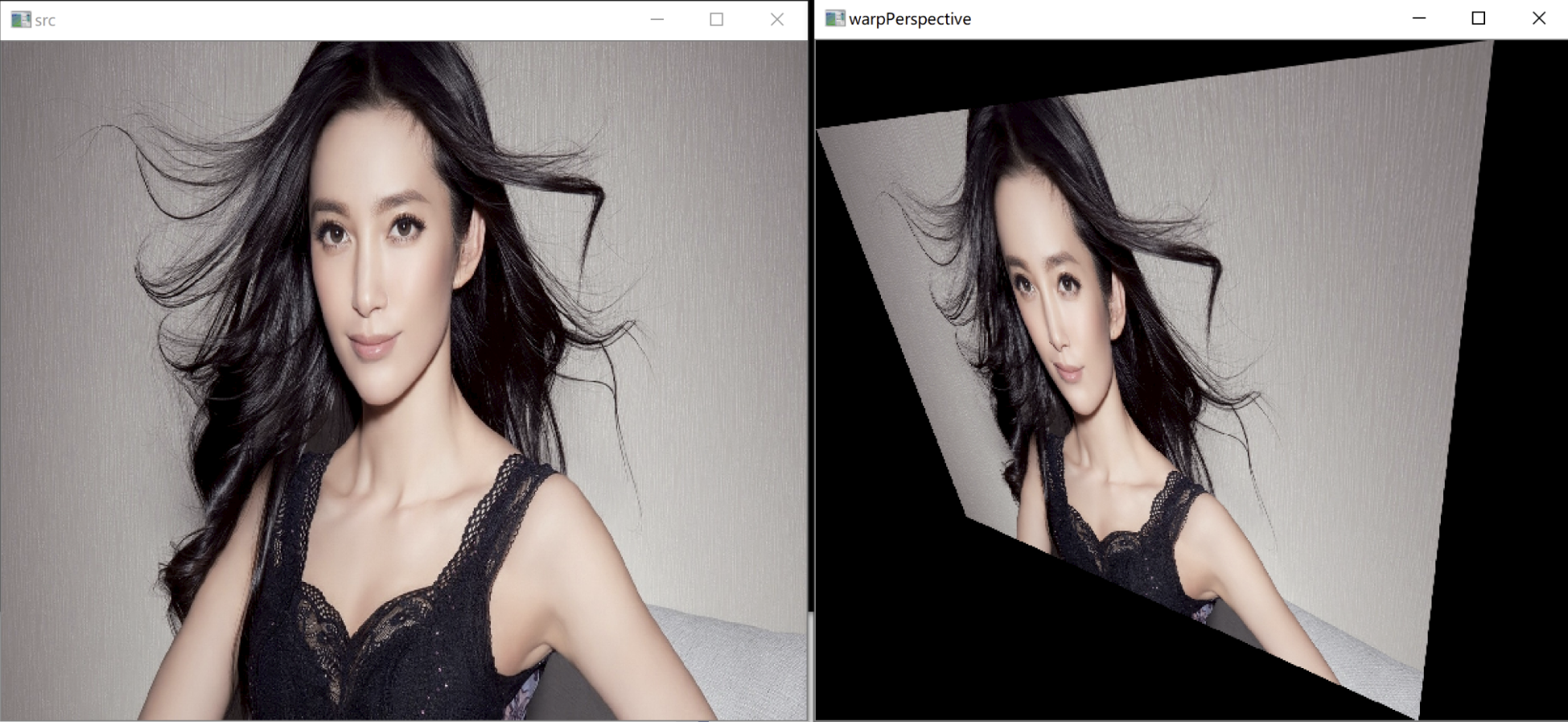4. 弯曲
4.1 S形状
int MPT_test_S_change(std::string file) {

double RANGE = 1000;
double PI = 3.1415926;

Mat dst(src.rows, src.cols, CV_8UC3, Scalar(0));
for (int i = 0; i < dst.rows; i++) {
double temp = (dst.cols - RANGE) / 2 + (dst.cols - RANGE) * sin((2 * PI * i) / dst.rows + PI) / 2;
for (int j = int(temp + 0.5); j < RANGE + temp; j++) {
int m = (int)((j - temp) * (src.cols) / RANGE);
if (m >= src.cols)
m = src.cols - 1;
if (m < 0)
m = 0;
dst.at<Vec3b>(i, j) = src.at<Vec3b>(i, m);
dst.at<Vec3b>(i, j) = src.at<Vec3b>(i, m);
dst.at<Vec3b>(i, j) = src.at<Vec3b>(i, m);
}
}
namedWindow("S形变形", CV_WINDOW_NORMAL);
imshow("S形变形", dst);
cv::waitKey(0);
return 0;
}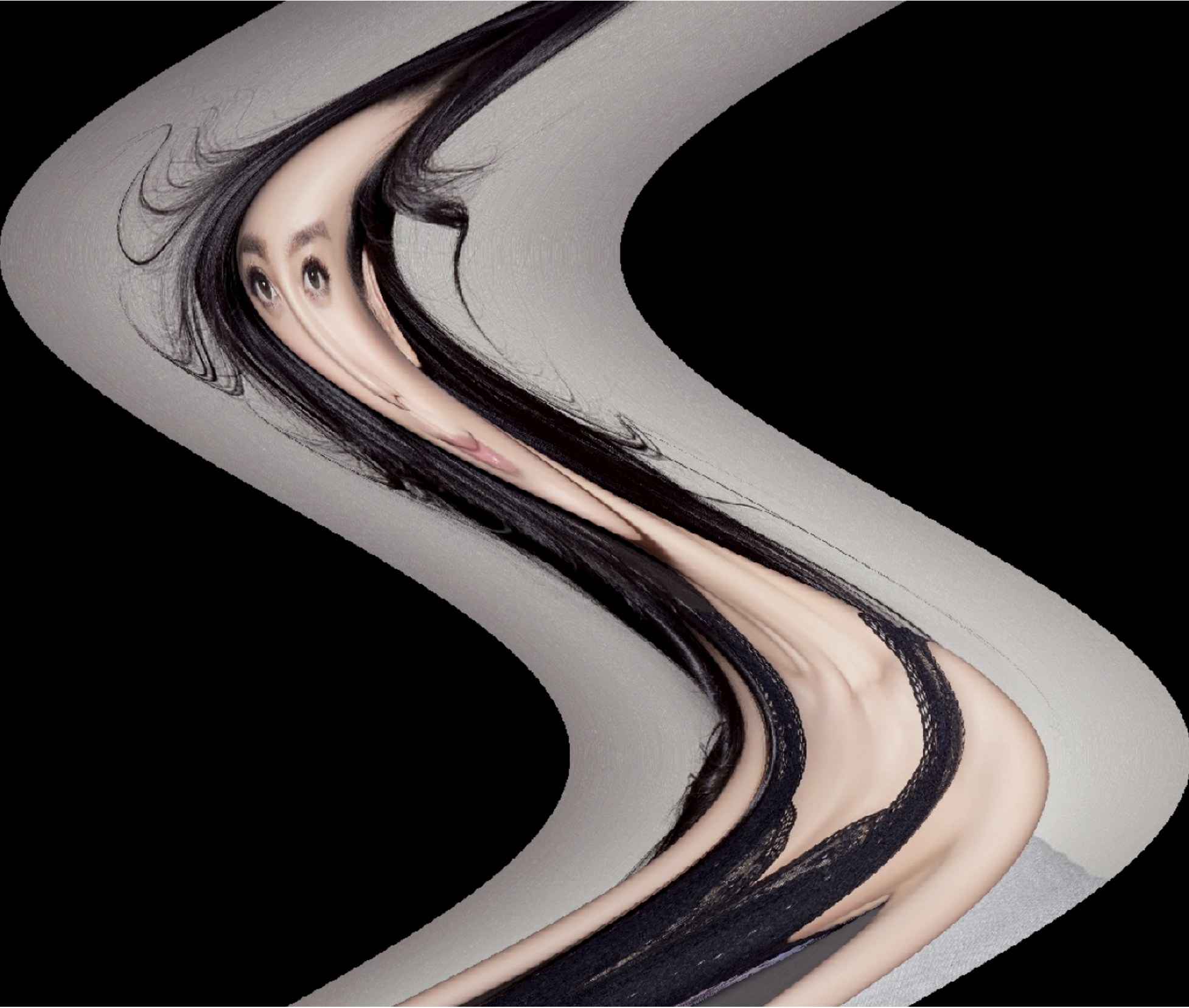4.2 波浪形
int MPT_test_2S_change(std::string file) {
double RANGE = 100;
double PI = 3.1415926;
Mat dst(src.rows, src.cols, CV_8UC3, Scalar(0));
for (int j = 0; j < dst.cols; j++) {
double temp = RANGE + RANGE * sin(j * PI * 2 / dst.cols);//利用sin函数得到一段正弦波，将图像映射到两个正弦波中即得到波浪形

for (int i = int(temp); i < dst.rows + temp - 2 * RANGE; i++) {
int m = (int)((i - temp) * (src.rows) / (dst.rows - RANGE));
if (m >= src.rows)
m = src.rows - 1;
if (m < 0)
m = 0;
dst.at<Vec3b>(i, j) = src.at<Vec3b>(m, j);
dst.at<Vec3b>(i, j) = src.at<Vec3b>(m, j);
dst.at<Vec3b>(i, j) = src.at<Vec3b>(m, j);
}
}
namedWindow("波浪变形", CV_WINDOW_NORMAL);
imshow("波浪变形", dst);
cv::waitKey(0);
return 0;
}5. 鱼眼特效
int MPT_test_fish_change(std::string file) {
double PI = 3.1415926;
cv::resize(src, src, Size(1080, 1080));
Mat dst(src.rows, src.cols, CV_8UC3, Scalar(0));

for (int id = 0; id < dst.rows; id++) {
for (int jd = 0; jd < dst.cols; jd++) {
double xd = jd * 2.0 / dst.cols - 1.0;
double yd = id * 2.0 / dst.cols - 1.0;
double rd = sqrt(xd * xd + yd * yd);
double phid = atan2(yd, xd);
double xs = asin(rd) * 2 / PI * cos(phid);
double ys = asin(rd) * 2 / PI * sin(phid);
int is = round((ys + 1.0) * dst.rows / 2.0);
int js = round((xs + 1.0) * dst.cols / 2.0);
if (is > dst.rows || is < 0 || js>dst.cols || js < 0)
continue;
dst.at<Vec3b>(id, jd) = src.at<Vec3b>(is, js);
dst.at<Vec3b>(id, jd) = src.at<Vec3b>(is, js);
dst.at<Vec3b>(id, jd) = src.at<Vec3b>(is, js);
}
}
cv::resize(dst, dst, Size(1080, 720));
cv::namedWindow("鱼眼特效", CV_WINDOW_NORMAL);
cv::imshow("鱼眼特效", dst);
cv::imwrite("../image/fisheye.jpg", dst);
cv::waitKey(0);
return 0;
}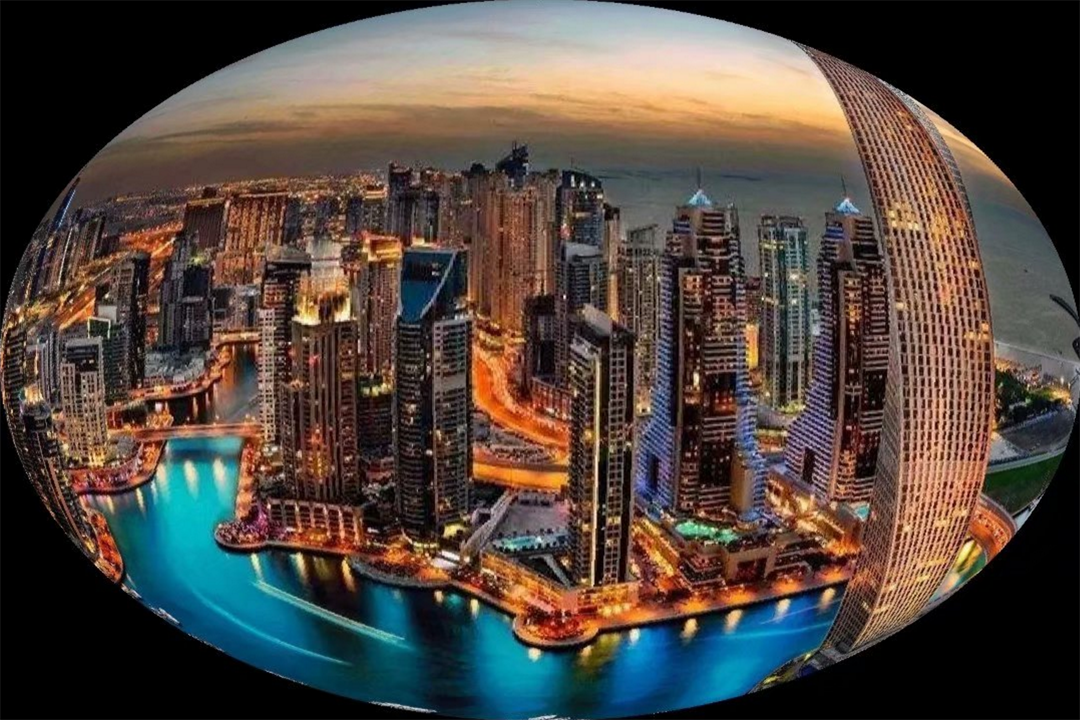6. 结构定义
enum BorderTypes {
BORDER_CONSTANT    = 0, //!< iiiiii|abcdefgh|iiiiiii  with some specified i
BORDER_REPLICATE   = 1, //!< aaaaaa|abcdefgh|hhhhhhh
BORDER_REFLECT     = 2, //!< fedcba|abcdefgh|hgfedcb
BORDER_WRAP        = 3, //!< cdefgh|abcdefgh|abcdefg
BORDER_REFLECT_101 = 4, //!< gfedcb|abcdefgh|gfedcba
BORDER_TRANSPARENT = 5, //!< uvwxyz|abcdefgh|ijklmno

BORDER_REFLECT101  = BORDER_REFLECT_101, //!< same as BORDER_REFLECT_101
BORDER_DEFAULT     = BORDER_REFLECT_101, //!< same as BORDER_REFLECT_101
BORDER_ISOLATED    = 16 //!< do not look outside of ROI
};

enum InterpolationFlags{
/** nearest neighbor interpolation */
INTER_NEAREST        = 0,
/** bilinear interpolation */
INTER_LINEAR         = 1,
/** bicubic interpolation */
INTER_CUBIC          = 2,
/** resampling using pixel area relation. It may be a preferred method for image decimation, as
it gives moire'-free results. But when the image is zoomed, it is similar to the INTER_NEAREST
method. */
INTER_AREA           = 3,
/** Lanczos interpolation over 8x8 neighborhood */
INTER_LANCZOS4       = 4,
/** Bit exact bilinear interpolation */
INTER_LINEAR_EXACT = 5,
/** mask for interpolation codes */
INTER_MAX            = 7,
/** flag, fills all of the destination image pixels. If some of them correspond to outliers in the
source image, they are set to zero */
WARP_FILL_OUTLIERS   = 8,
/** flag, inverse transformation

For example, #linearPolar or #logPolar transforms:
- flag is __not__ set: \f$dst( \rho , \phi ) = src(x,y)\f$
- flag is set: \f$dst(x,y) = src( \rho , \phi )\f$
*/
WARP_INVERSE_MAP     = 16
};

结构定义（中文）
enum InterpolationFlags {
/**最近邻插值*/
INTER_NEAREST = 0，
/**双线性插值*/
INTER_LINEAR = 1
/**双三次插值*/
INTER_CUBIC = 2
/**使用像素面积关系重新采样。它可能是图像抽取的首选方法，因为它提供无云纹的结果。但是当图像放大时，它类似于INTER_NEAREST方法。 */
INTER_AREA = 3，
/**在8x8邻域上进行Lanczos插值*/
INTER_LANCZOS4 = 4
/**位精确双线性插值*/
INTER_LINEAR_EXACT = 5
/**内插代码的掩码*/
INTER_MAX = 7
/**标志，填充所有目标图像像素。如果其中一些对应于源图像，它们设置为零*/
WARP_FILL_OUTLIERS = 8
/** 标志，逆变换例如，#linearPolar或#logPolar转换为：-__not__标志未设置：\ f $dst（\ rho，\ phi）= src（x，y）\ f$-设置了标志：\ f $dst（x，y）= src（\ rho，\ phi）\ f$ */
WARP_INVERSE_MAP = 16
};

参考文档
参考文档


展开全文opencv
• 仿射空间与仿射变换 一个简单的几何问题 已知AC∥BD∥FEAC \parallel BD \parallel FEAC∥BD∥FE 另外AC,BD,AF,FB长度分别为n,m,a,b，求FE的长度. 求解： 作辅助线CB交于EF于点G 在△ABC\triangle{ABC}△ABC与△DBC...
仿射空间与仿射变换
一个简单的几何问题已知$AC \parallel BD \parallel FE$
另外AC,BD,AF,FB长度分别为n,m,a,b，求FE的长度.
求解：
作辅助线CB交于EF于点G
在$\triangle{ABC}$与$\triangle{DBC}$中，
根据三角形相似，容易求得
$GF = n \cdot \frac{b}{a+b}$
$GE = m \cdot \frac{a}{a+b}$
$FE = GF + GE = n \cdot \frac{b}{a+b} + m \cdot \frac{a}{a+b}$
也可以写成：
$FE = n \cdot \frac{DE}{CD} + m \cdot \frac{EC}{CD}$
值得注意的是，n与m的系数之和为1, 这一点非常重要.
A与B重合时，即ABCD在同一条直线上时，AC可看成C点的坐标值$y_1$，BD可看成D点坐标值$y_2$.
E点的坐标y，即FE同样适用上面公式：
$y = y_1 \cdot \frac{DE}{CD} + y_2 \cdot \frac{EC}{CD}$
显然，这里不限于纵坐标，换成x也成立.
容易推导，C,D为平面任意向量$\vec{C}$,$\vec{D}$时
$\vec{E} = \frac{b}{a+b} \cdot \vec{C} + \frac{a}{a+b} \cdot\vec{D}$
回到最开始的情况，如果把条件返过来，
如果（a与b比例为任意值）：
$FE = n \cdot \frac{b}{a+b} + m \cdot \frac{a}{a+b}$
那么能证明E在CD的连线上吗？
当然可以，而且比较明显，这里不再证明.
于是，我们得出一个重要结论（1），即平面上的两点A，B，它们连接上的点P可以表示为
$\vec{P} = a\vec{A} + b\vec{B}$
其中 $a+b = 1$
溶液混合问题
在做进一步推导之前，我们顺带解决一个溶液混合问题.
问题：两种浓度不同的溶液（比如盐水），X浓度为A，Y浓度为B，要配制成浓度为C的浓度，求X与Y的配比.
求解：
假设X的用量是a，Y的用量为b，则混合后的浓度为：
$C = \frac{A a + B b}{a + b}$
显然这个问题是可以归结为上述的几何问题的.上述问题等价于，
已经EF 与 n , m, 求a与b的比例.
作HI平行于AB，交BD于I，交AC延长线于点H.
令EF为l.
$\frac{a}{b} = \frac{CE}{ED} = \frac{CH}{ID} = \frac{l-n}{m-l}$
溶液的问题,BD相当于浓度A，CA相当于浓度B，EF相当于浓度C，所以答案是：
$\frac{a}{b} = \frac{B-C}{C-A}$
推广到二维平面由上述结论（1）
参数,a,b,c,d,满足：
$a + b = 1, c + d = 1$
$\vec{D} = a\vec{A} + b\vec{B}$
$\vec{E} = d\vec{D}+c\vec{C}$
$\vec{E} = d(a\vec{A}+b\vec{B})+c\vec{C} \vec{E} = da\vec{A}+db\vec{B}+c\vec{C}$
$da+db+c = d(a+b) + c = d+c = 1$
即平面上的任意一点都可以用
$\vec{A},\vec{B},\vec{C}$表示，它们系数之和为1.
依此类推，不仅可能表示平面上的点，三维及更高维的情形下，都有这样的规律.
反过来，只要系数之和为零，这个点就一定在平面上吗？
答案是的.
假设
$\vec{P} = a\vec{A}+b\vec{B}+c\vec{C}$
$a+b+c = 1$
那么P一定在ABC平面上.
证明：
$a\vec{A}+b\vec{B}+c\vec{C}$
$= (1-c) \frac{1}{1-c} (a\vec{A}+b\vec{B})+c\vec{C}$
$= (1-c) \frac{1}{a+b} (a\vec{A}+b\vec{B})+c\vec{C}$
$= (1-c) (\frac{a}{a+b} \vec{A}+\frac{b}{a+b}\vec{B})+c\vec{C}$
显然
$\frac{a}{a+b} \vec{A}+\frac{b}{a+b}\vec{B}$
表示AB直线的上一点，这里用P表示，上式可写成：
$a\vec{A}+b\vec{B}+c\vec{C} = (1-c) \vec{P}+c\vec{C}$
显然$(1-c) \vec{P}+c\vec{C}$,两向量系数之和为1，即表示PC直线上的一点，所以这个点在ABC平面上.
如果使用平面n个顶点，坐标为$P_1,P_2,P_3,...,P_n$
那么
$\lambda_1 P_1+ \lambda_2 P_2+ \lambda_3 P_3+ ... + \lambda_n P_n$
表示怎样一个图形呢？
其中$\lambda_1 + \lambda_2 + \lambda_3 + ... + \lambda_n = 1$
且$\lambda_1 , \lambda_2 , \lambda_3 , ... , \lambda_n$不小于0
答案是,一个凸多边形, 一个包含这n个点的最小凸多边形.
根据上面的推导，这个图形是从两点构造线段开始的，再以线段上的某一点与下一个顶点构造直接，即形成一个三角形，
往后，在三角形上取一个点与下一点构造，这样的方法只会使整个多边形扩大，而且满足凸多边形性质.
仿射空间与仿射组合
仿射这个词，从字面上是看不出其对应的含义的，甚至这个词五笔都不支持连打.
对应的英文为affline，同样也看不明白它的含义.不清楚为什么要翻译成仿射.
下面抛开这个词，这里描述这样一些实事：
实事1
所有矩阵变换都可以转换成基变换，当然所有基变换也都可以转换成矩阵变换.
具体参考文章《坐标轴变换与坐标变换》
显然，再怎么拉扯坐标轴，都不会影响到物体的平行关系与比例关系.
这样的变换称为线性变换，用Y = MX表示，这个是我们熟知的.
实事2
用以下向量为基，
$\vec{P_1},\vec{P_2},\vec{P_3},...,\vec{P_n}$
通过这个组合
$\lambda_1 \vec{P_1}+ \lambda_2 \vec{P_2}+ \lambda_3 \vec{P_3}+ ... + \lambda_n \vec{P_n} \quad (\lambda_1 + \lambda_2 + \lambda_3 + ... + \lambda_n = 1)$
构成的空间，这里暂时称之为阿法莱空间（随意取的代称，没有为什么）.
其描述向量所用的组合，自然称为阿法莱组合.
这个空间有一个特点，
就是不管坐标系如何变换，即线性变换，甚至包括移动坐标系原点，都不影响组合的写法.
这个变换是线性变换基本上加上用于修改原点的平移，可以写成：
Y = MX + B
我们把这样的变换称之为阿法莱变换.根据实事一，这个变换同样不会影响平行关系与比例关系.
这个阿法莱没什么含义，先贤们已经给它取了一个比较能描述其性质的名字，英文为affline,中文译为仿射.
于是有仿射空间，仿射组合与仿射变换.
参考
Affine combination
《坐标轴变换与坐标变换》


展开全文• 仿射变换 一个任意的仿射变换都能表示为 乘以一个矩阵 (线性变换) 接着再 加上一个向量 (平移). 综上所述, 我们能够用仿射变换来表示: 旋转 (线性变换) 平移 (向量加) 缩放操作 (线性变换) ...计算机视觉
• ## 仿射加密法

万次阅读 2020-02-19 21:42:03
仿射加密法 乘数加密法 + 凯撒加密法 = 仿射加密法 凯撒加密法：[https://blog.csdn.net/youngdianfeng/article/details/104333494] 乘数加密法下面介绍。 乘数加密法python
• 语言：python3 实验目的： 通过编程实现代换密码算法——仿射密码和简单置换密码算法，加深对古典密码体制的了解，为深入学习密码学奠定基础。 实验内容： ... 仿射密码加密解密模块 @author WQ @.python
• 仿射函数，即最高次数为1的多项式函数，是基于向量的运算 常数项为零的仿射函数称为线性函数，基于单个数字的运算 线性函数是过原点的仿射函数 仿射函数即由 1 阶多项式构成的函数，一般形式为 f(x)=Ax+b，这里，...
• 证明了李安民教授等于2000年构造的具给定仿射Gauss-Kronecker曲率的超曲面一定是仿射完备这一猜测．
• 基于Visual Studio的Opencv进行图像的仿射变换，通过构建仿射矩阵来旋转图像。图像处理
• ## 仿射空间

千次阅读 2019-09-27 14:47:16
直观理解仿射空间 向量空间（也叫线性空间）我们是很熟悉的，那么可以借助向量空间给仿射空间一个直观的定义：仿射空间就是没有原点的向量空间。 向量空间没有了原点，会有什么样的影响？向量是基于原点的，向量有...
• 如何通俗地讲解「仿射变换」这个概念？ - 马同学的回答 - 知乎 https://www.zhihu.com/question/20666664/answer/157400568 https://www.wikiwand.com/zh/%E4%BB%BF%E5%B0%84%E5%8F%98%E6%8D%A2 ...
• 仿射变换（Affine transformation），又称仿射映射，是指在几何中，对一个向量空间进行一次线性变换并接上一个平移，变换为另一个向量空间。 它是一种二维坐标到二维坐标间的线性变换，保持了二维图形的“平直性”...计算机视觉 算法...